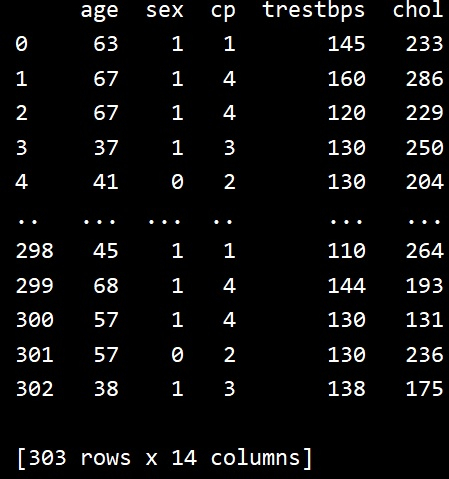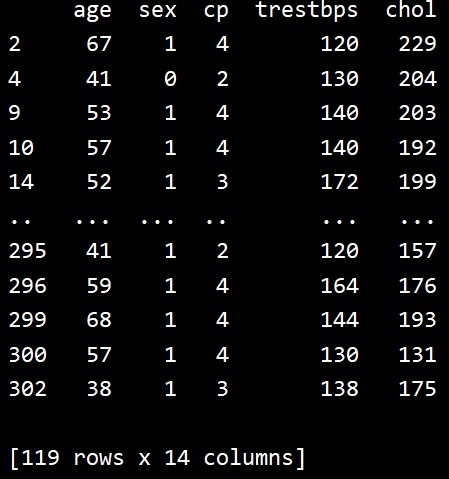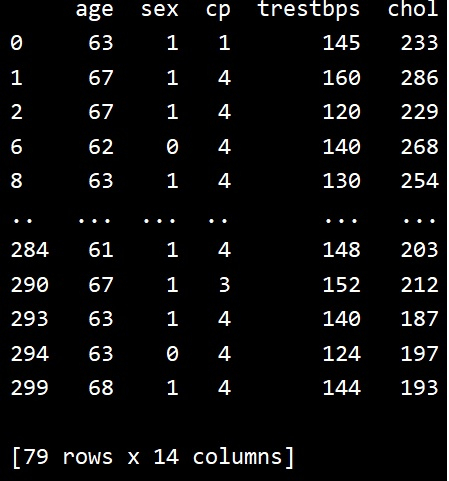# Python - Filtering data with Pandas .query() method

Pandas is a very widely used python library for data cleansing, data analysis etc. In this article we will see how we can use the query method to fetch specific data from a given data set. We can have both single and multiple conditions inside a query.

Let’s first read the data into a pandas data frame using the pandas library. The below program just does that.

## Example

import pandas as pd

# Reading data frame from csv file

print(data)

## Output

Running the above code gives us the following result −## Query with single condition

Next we see how we can use the query method with single condition. As you can see only 119 rows from the original 303 rows are returned as a result.

## Example

import pandas as pd

# Data frame from csv file

data.query('chol < 230', inplace=True)

# Result
print(data)

## Output

Running the above code gives us the following result −## Query with multiple conditions

In a similar approach as above we can apply multiple conditions to the query method. This will restrict the result data set further. Only 79 rows are returned now when we also restrict the age to greater than 60.

## Example

import pandas as pd

# Data frame from csv file

data.query('chol < 230' and 'age > 60', inplace=True)

# Result
print(data)

## Output

Running the above code gives us the following result −Updated on: 22-Jul-2020

2K+ Views